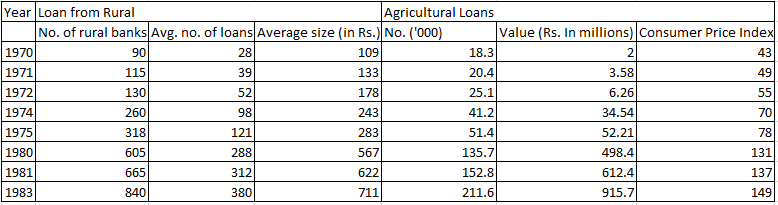### CAT 1997 Question Paper Question 26

Instructions

The table given below gives the annual details of loans from rural banks and agricultural loans over the years 1970 to 1983. Using this data answer the questions that follow.Question 26

# What is the value(in millions) of the agricultural loans in 1983 at 1970 prices?

Solution

To change the prices from one year to another year, we have to use the Consumer Price Index.

Value in 1983 = 915.7 millions

CPI in 1983 = 149

CPI in 1970 = 43

Required value in 1970 = $$\frac{43}{149}*915.7$$ = Rs 264 million